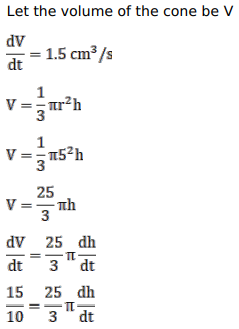# An inverted cone has a depth of

Question:

An inverted cone has a depth of $40 \mathrm{~cm}$ and a base of radius $5 \mathrm{~cm}$. Water is poured into it at a rate of $1.5$ cubic centimetres per minute. Find the rate at which the level of water in the cone is rising when the depth is $4 \mathrm{~cm}$.

Solution: